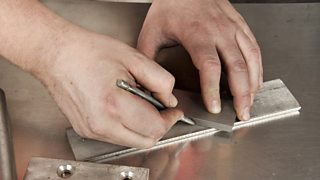# Marking out

Accuracy is vital to a quality finished product. The UK recommends the use of metric measurements using millimetres (mm), centimetres (cm) and metres (m).

## Datum references

When an object, are used as a reference point for all measurements to be taken from and can be chosen by the manufacturer. By taking measurements from a small number of set points, accuracy can be improved and maintained.

A datum reference can be along the straight edge of a design, known as datum surface, or a single datum point, often found in the corner of a design. In a circular shape, it would be around the edge or from the centre.

In this example, the opposite corners of the shape are connected with and the intersection creates a reference point in the centre of the work. This is one example of a datum point.

Datum references are particularly useful for aligning machines, which use the point where the X- and Y-axis meet as a datum reference. They can also be used to sizes up and down in measurements by using the X- and Y-axis.

Working out reference points in a square shape is simple but most objects are more complex.

## Calculating angles

The use of trigonometry can be used to work out accurate angles:

• sin = opposite ÷ hypotenuse
• cos = adjacent ÷ hypotenuse
• tan = opposite ÷ adjacent
The formula used will depend on the information given.

### Example

The height of the triangular section below is calculated using trigonometry:

We have the angle and the adjacent side and are looking for the opposite side. So from the above formulas the best choice is:

Calculate the tangent of 50° using a calculator:

tan (50) = 1.19175359

Multiply by 100 mm (the adjacent) to get the height:

1.19175359 × 100 = 119.175359

= 119 mm

This can then be used to calculate the area:

Area of a triangle = 12 (height × width)

= 12 (119 mm × 100 mm) = 12 (11,900 mm2)

= 5,950 mm2 or 595 cm2

A protractor can be used to measure angles accurately during marking out.

Question

A bracket is required for a new shop sign. What is the size of angle x?

tan (x) = opposite ÷ adjacent

tan (x) = 8 ÷ 4 = 2

tan (x) = 2

x = tan-1 (2)

x = 60°

## Orthographic drawing

An orthographic drawing shows the measurements on each surface of an object. They would be used to help a manufacturer make a product accurately.

On this orthographic drawing, one example of a datum reference could be the centre line of the hole, and all measurements could be taken from this before cutting begins

Where the hole would appear if it were shown in a 3D drawing (third angle orthographic projection):

## Marking-out tools

are tools made specially to help place a material in the same place repeatedly. They are particularly useful for marking out where holes need to be drilled or joints cut. They cut production costs by saving waste, speeding up the process and improving accuracy.

A range of marking-out tools can be used to show where to cut, drill and assemble parts. Simple marking out can be done with a pencil, but more accurate and consistent markings can be done with:

• tape measure - measure straight and curved shapes
• pins - hold pattern pieces in place and mark points on fabric
• craft knife - cut and score (mark), particularly used on paper and card
• compass - mark out circles
• - a sharp point to put a fine scratch on the surface of the metal that is being marked
• mitre square - measure 45 degree and 135 degree angles for mitre joints in picture frames
• mortise gauge - mark out lines parallel to an edge
• - used to create a drill point before a hole is drilled so that the drill bit does not slip on the surface
• or try square - used for marking out at 90 degrees to an edge or face
• marking knife - score lines
• sliding bevel - can be adjusted to mark a specific angleScriber

Surface patterns on paper and fabric mean that marking out should take into account a repeated pattern to make sure the images match up - marking out before cutting can help minimise waste. Paper pattern pieces are used to cut out garment pieces in fabric accurately - they carry symbols and markings to show the direction of the and have to show where they attach to another piece.